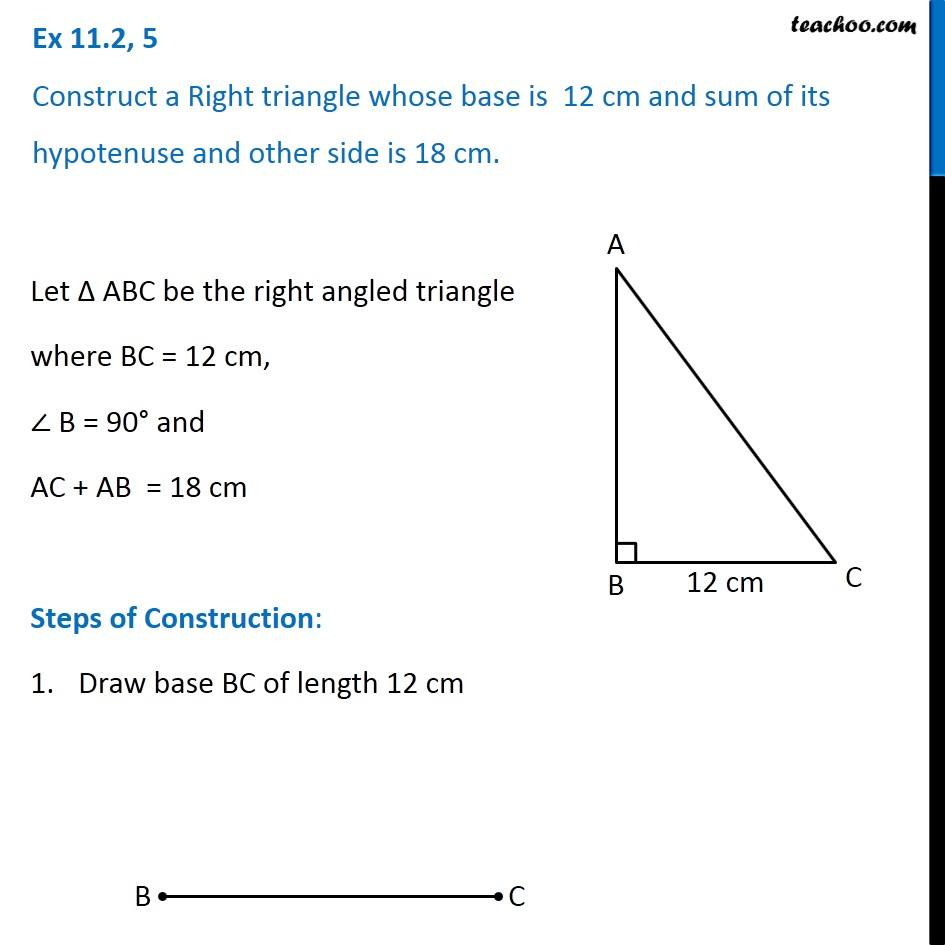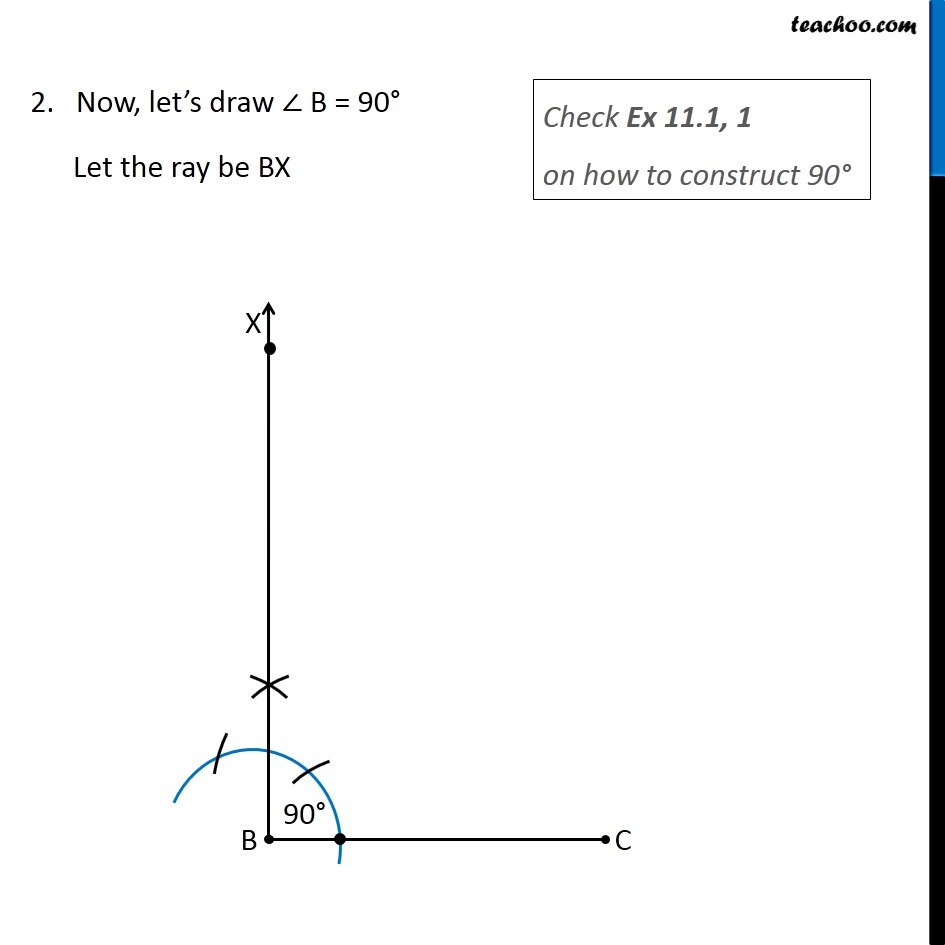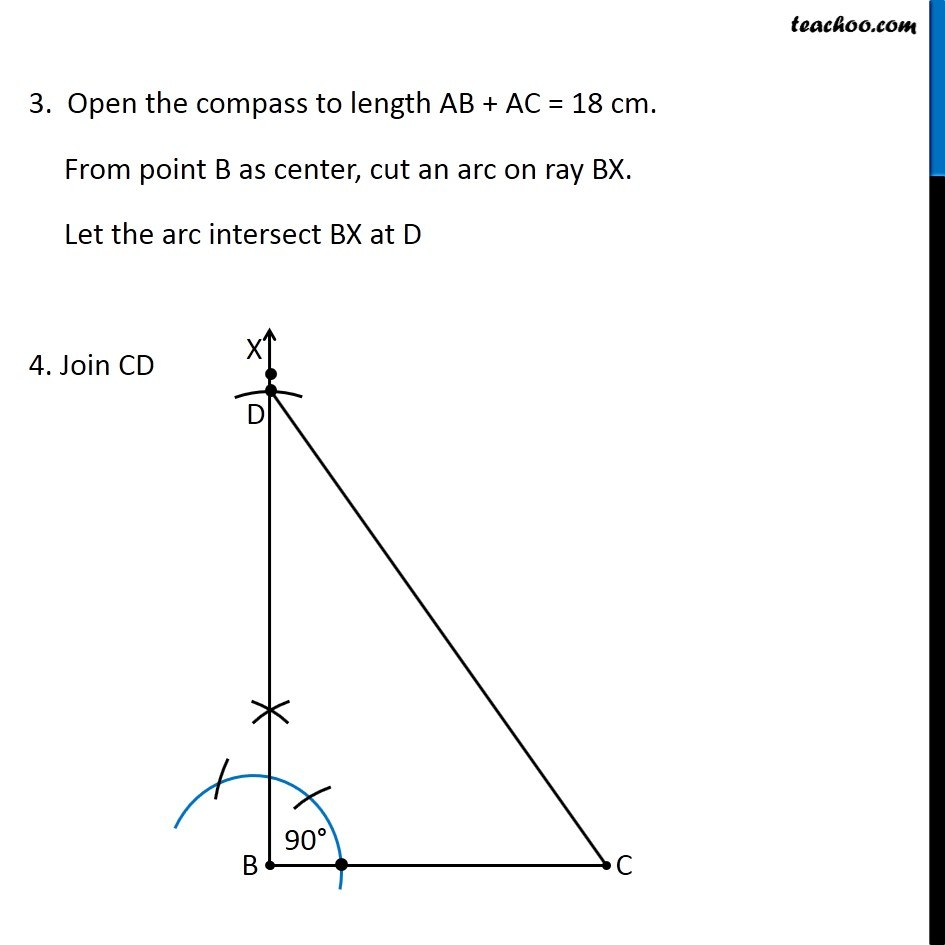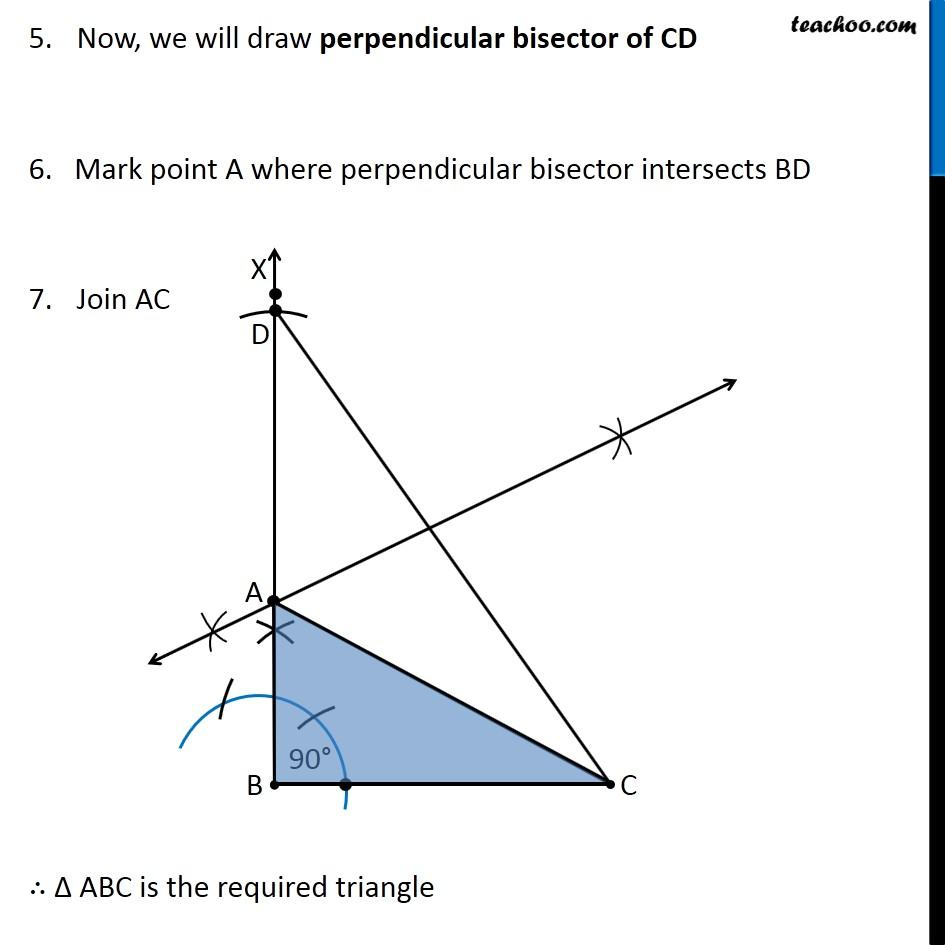1. Class 9
2. Important Questions for Exam - Class 9
3. Chapter 11 Class 9 Constructions

Transcript

Ex 11.2, 5 Construct a Right triangle whose base is 12 cm and sum of its hypotenuse and other side is 18 cm. Let Δ ABC be the right angled triangle where BC = 12 cm, ∠ B = 90° and AC + AB = 18 cm Steps of Construction: Draw base BC of length 12 cm 2. Now, let’s draw ∠ B = 90° Let the ray be BX Check Ex 11.1, 1 on how to construct 90° 3. Open the compass to length AB + AC = 18 cm. From point B as center, cut an arc on ray BX. Let the arc intersect BX at D 4. Join CD 3. Open the compass to length AB + AC = 18 cm. From point B as center, cut an arc on ray BX. Let the arc intersect BX at D 4. Join CD

Chapter 11 Class 9 Constructions

Class 9
Important Questions for Exam - Class 9